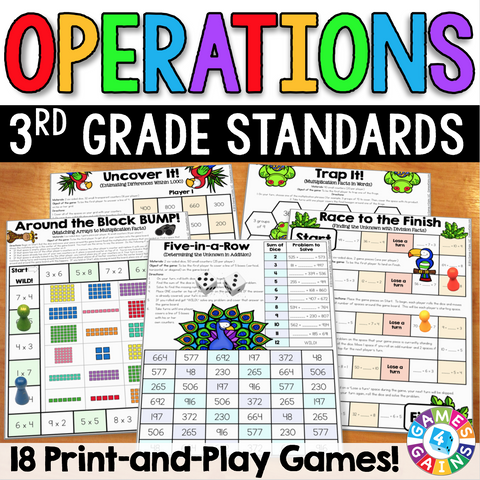• \$595

These games make 3rd grade operations standards so much fun to practice! This 3rd Grade Operations Games Pack contains 18 fun and engaging printable board games to help students to practice 3rd grade addition, subtraction, multiplication, and division standards.

This pack includes DIFFERENTIATED games that cover the following 3rd grade operations skills:

• Subtracting numbers within 1,000
• Multiplying basic facts within 100
• Dividing basic facts within 100
• Multiplying by multiples of 10

These games are so simple to use and require very minimal prep - just print, grab some basic materials, and play! They are perfect to use in math centers or as extension activities when students complete their work!

Included with this 3rd Grade Operations Games Pack:
• Instructions for play
• 18 printable games
• Answer keys for all of the games

These 3rd Grade Operations Games support the following Common Core math standards:

CCSS.MATH.CONTENT.3.OA.A.1
Interpret products of whole numbers, e.g., interpret 5 × 7 as the total number of objects in 5 groups of 7 objects each.

CCSS.MATH.CONTENT.3.OA.A.2
Interpret whole-number quotients of whole numbers, e.g., interpret 56 ÷ 8 as the number of objects in each share when 56 objects are partitioned equally into 8 shares, or as a number of shares when 56 objects are partitioned into equal shares of 8 objects each.

CCSS.MATH.CONTENT.3.OA.A.4
Determine the unknown whole number in a multiplication or division equation relating three whole numbers. For example, determine the unknown number that makes the equation true in each of the equations 8 × ? = 48, 5 = _ ÷ 3, 6 × 6 = ?

CCSS.MATH.CONTENT.3.OA.C.7
Fluently multiply and divide within 100. By the end of Grade 3, know from memory all products of two one-digit numbers.

CCSS.MATH.CONTENT.3.NBT.A.2
Fluently add and subtract within 1000 using strategies and algorithms based on place value, properties of operations, and/or the relationship between addition and subtraction.

CCSS.MATH.CONTENT.3.NBT.A.3
Multiply one-digit whole numbers by multiples of 10 in the range 10-90 (e.g., 9 × 80, 5 × 60) using strategies based on place value and properties of operations.